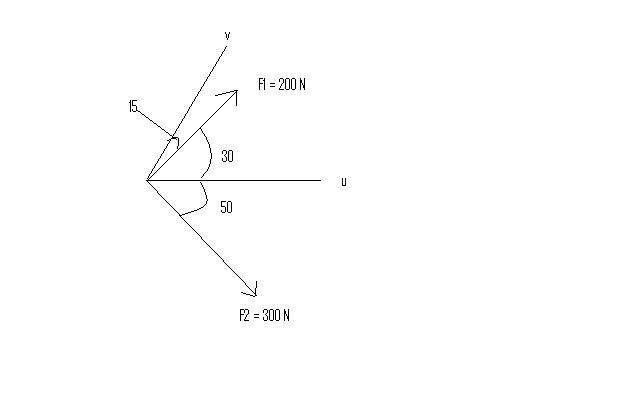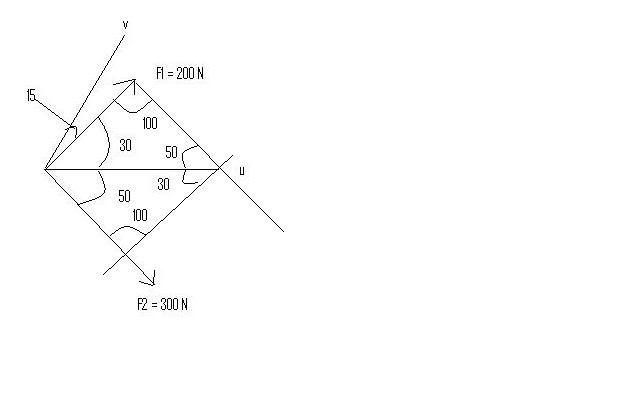# Vector Problem-Direction and Magnitude

## Homework Statement

Determine the magnitude of the resultant force Fr=F1+F2 and its direction measured from the positive u axis.Law of Sines?

## The Attempt at a Solution

I drew parallel lines and attempted to use the sine law, but I got two different answers.
Here's the equations I used:
Fr/sin100 = 200/sin50
Fr/sin100 = 300/sin30Using these equations, I got two different answers for Fr, and didn't get any direction.

Other than what I tried, I don't know what to do. We weren't taught how to find the magnitude from components or taught how to find direction.

#### Attachments

Last edited:

Yes, sir.

learningphysics
Homework Helper
The problem is that you're assuming F1 + F2 ends up along the u axis... it doesn't.

I have to use the angles to figure out which direction the resultant force is pointing after $$F_1$$ and $$F_2$$ are added, but I don't know how.

learningphysics
Homework Helper
The things you know for sure are the 200, 300 sides... and the 100 degrees angle. You can use those to get the magnitude of F1+F2, and the angles....

Draw a triangle separately from the axes... when you get the angles... then you can draw F1+F2 in correctly...

Ok, thank you sir.